8+ Sample Metric System Conversion Chart Templates

Although different measurements and the definitions of metric conversion units have been revised, the official system of measurements of most countries is the modern form of the metric system which is known as the ‘International System of Units’. There are other metric systems followed by people living in the US and the UK. Therefore, the Metric Conversion Chart Templates not only assists in converting the various units of measurement but also helps in understanding the various alternate systems of metric conversions.

Metric System Table Conversion Chart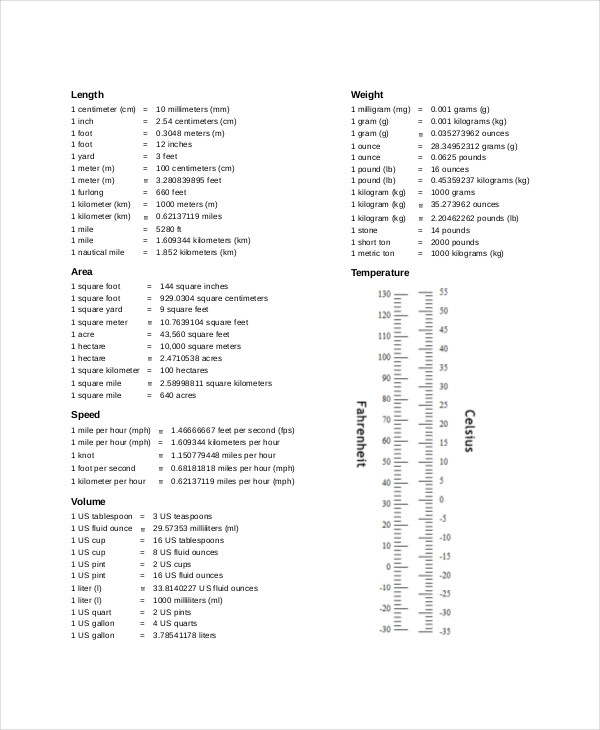quizzes.cc

The Metric System table conversion Chart Templates assists in the conversion of various units of measurement such as meter to foot, centimeters to inches, currency conversions and a number of other quantities.

Metric System Unit Conversion Chart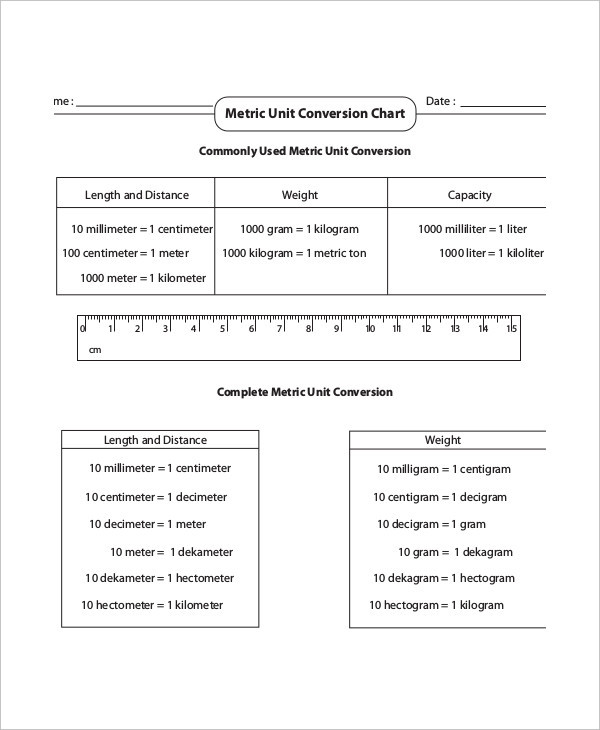mathworksheets4kids.com

This conversion chart is used for length, weight, temperature, area and distance conversions and finds much utility in our daily lives. The Metric System Unit Conversion Chart makes complex mathematics absolutely unnecessary.

Metric System Length Conversion Chart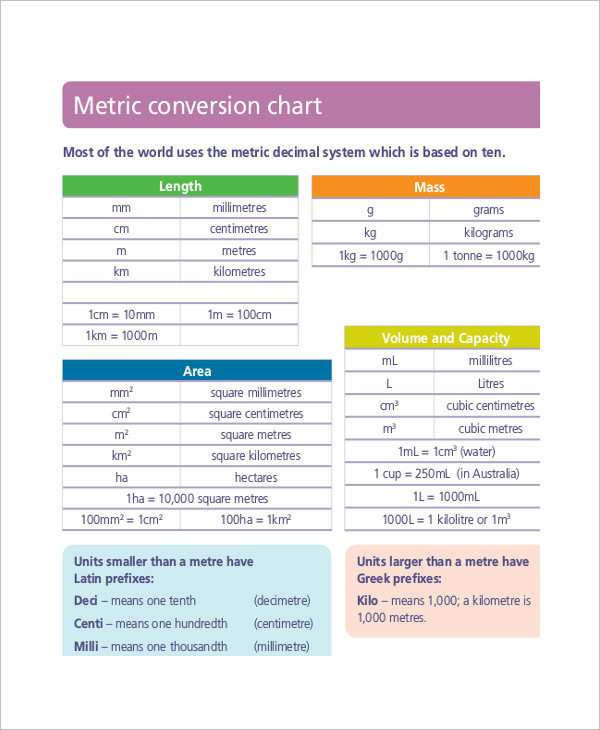det.nsw.edu.au

The Metric System Length Conversion Chart is exclusively used to convert only units of length such as millimeters, centimeters, meters and kilometers into inches, feet, yards, mile and league.

Metric System Weight Conversion Chart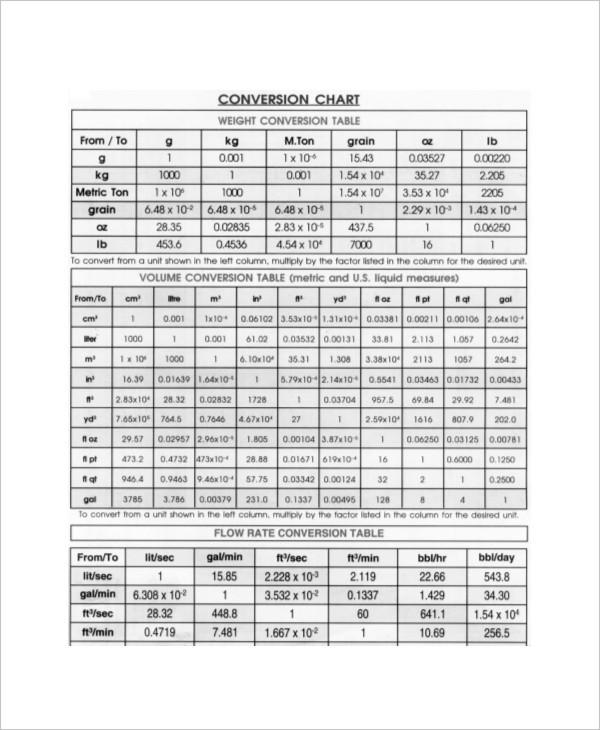blulux.com

The Metric System Length Conversion Chart is exclusively used to convert only units of weight such as kilogram, hectogram, metric ton and quintal which can save you the hassle of making complex calculations.

Nursing Metric System Conversion Chart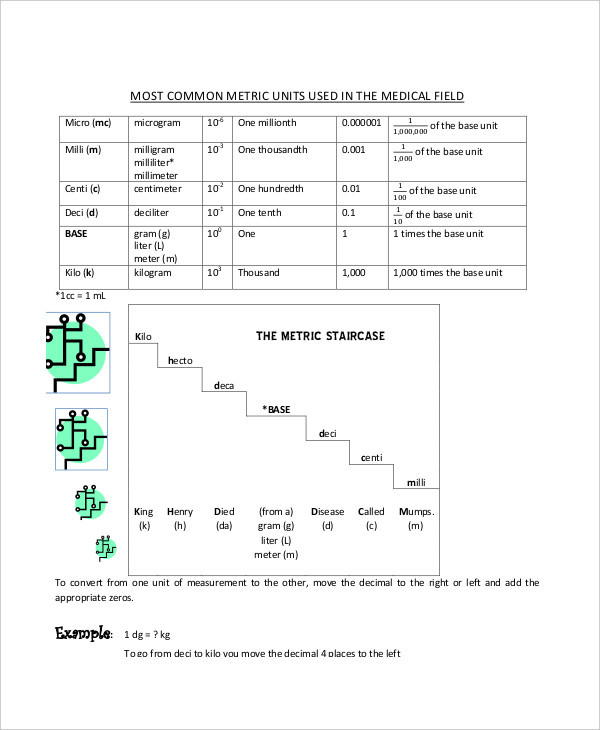laniertech.edu

Nurses require referring to the metric system conversion chart for multiple reasons such as measuring food and medicine dosage for the patients and keeping a tab of the total count of calories to maintain their patient’s etc

Metric System Conversion Chart Worksheet Example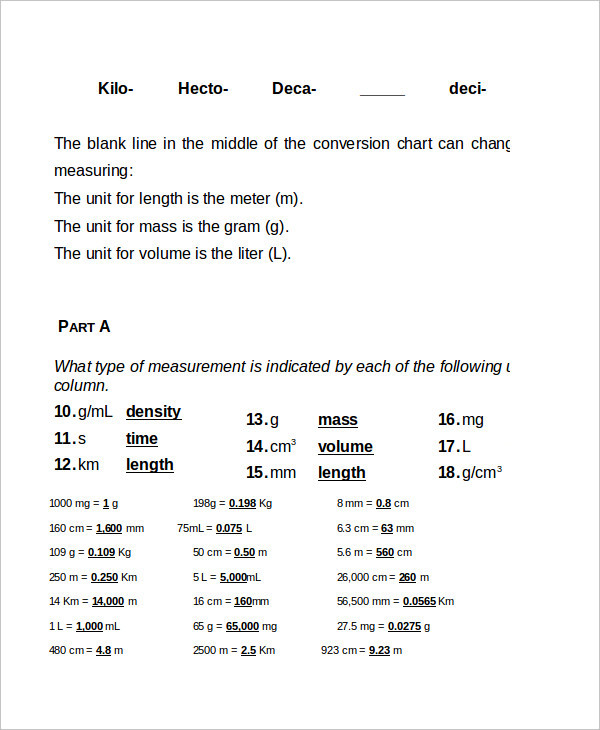trinitypride.org

Sample Metric System Conversion Chart Template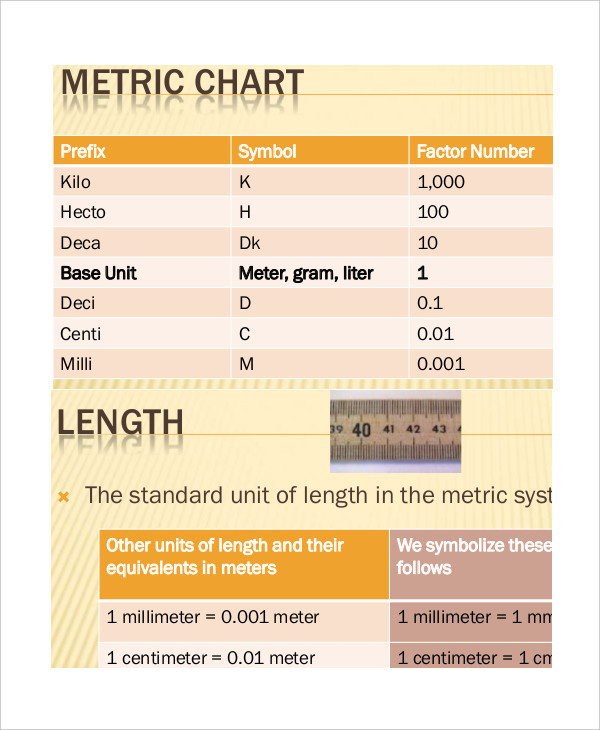sradai.tripod.com

Simple Metric System Conversion Chart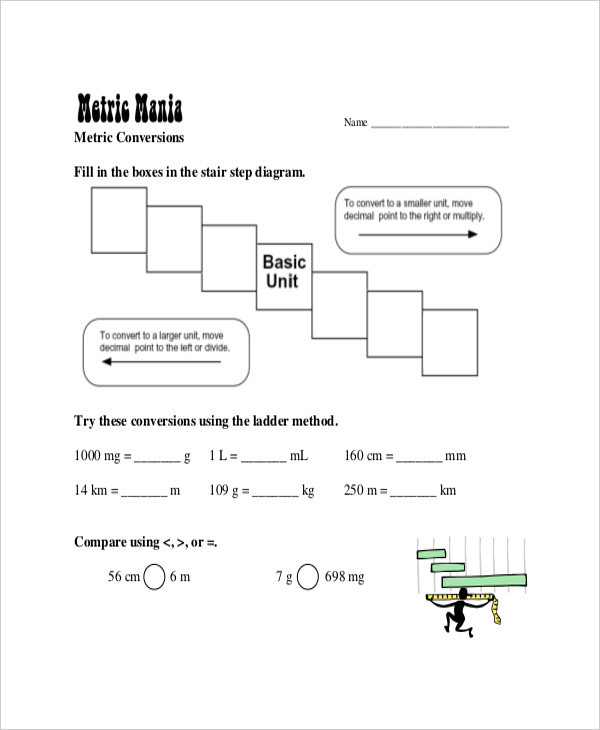sciencespot.net

> How to use the Metric System Conversion Chart?

If you want to travel around the world, study science and mathematics or use simple conversion units of measurement in your daily endeavors to make life easy, it is imperative to understand the metric system. Sometimes understanding the simplest of conversions such as length, volume and mass can be quite handy and helpful. You can also see Normal Height And Weight Chart Templates.

Therefore, various forms of customized Metric System conversion charts have been exclusively created for your professions so that you are able to derive the results in the least possible time without having to perform complex mathematical calculations yourself. All you have to do is print this and hang it where you can see or simply save a copy on your desktop.

> What are the benefits of the Metric System Conversion Chart?

With the help of the Metric system conversion chart, the goal is to learn to use metric units in a natural way. There are millions of people in the world who don’t know any algebra at all but knows what a kilogram of rice is, or how much is a liter of kerosene.

There could be plenty of occasions where you would not right away know or understand a form of measurement you hear or read. You might be unsure of what units are to be used to describe a certain quantity. In such situations, the utility of the Metric System conversion chart comes handy. You can also see Height And Weight Chart Templates For Kid.

Learning metric system through the Metric Conversion charts can be a simple exercise of referring to it and allowing it to do the complicated calculations for you. If you are looking to create a customized metric system conversion chart you can seek help from the editable and downloaded templates available at template.net.